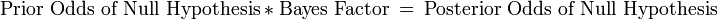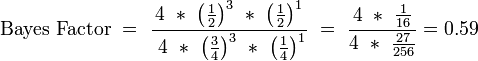# Statistical significance

In statistics, statistical significance is a "term indicating that the results obtained in an analysis of study data are unlikely to have occurred by chance, and the null hypothesis is rejected. When statistically significant, the probability of the observed results, given the null hypothesis, falls below a specified level of probability (most often P < 0.05)." The P-value, which is used to represent the likelihood the observed results are due to chance, is defined at "the probability, under the assumption of no effect or no difference (the null hypothesis), of obtaining a result equal to or more extreme than what was actually observed."

## Hypothesis testing

Usually, the null hypothesis claims that there is no difference between two samples in regard to the factor being studied.

### Choosing a statistical method

The choice of statistical method to use in an analysis is determined by:

• Type of data, for example: continuous, categorical, dichotomous
• Whether the data is normally distributed. Various normality tests such as the Shapiro-Wilk are available.
• Whether the samples are independent or paired
• Number of samples to compare

## Statistical errors

Two errors can occur in assessing the probability that the null hypothesis is true:

### Type I error (alpha error)

Type I error, also called alpha error, is the rejection of a correct null hypothesis. The probability of this is usually expressed by the p-value. Usually the null hypothesis is rejected if the p-value, or the chance of a type I error, is less than 5%. However, this threshold may be adjusted when multiple hypotheses are tested.

### Type II error (beta error)

Type II error, also called beta error, is the acceptance of an incorrect null hypothesis. This error may occur when the sample size was insufficient to have power to detect a statistically significant difference.

## Philosophical approaches to error testing

### Frequentist method

This approach uses mathematical formulas to calculate deductive probabilities (p-value) of an experimental result. This approach can generate confidence intervals.

A problem with the frequentist analyses of p-values is that they may overstate "statistical significance".

### Likelihood or Bayesian method

Some argue that the P-value should be interpreted in light of how plausible is the hypothesis based on the totality of prior research and physiologic knowledge. This approach can generate Bayesian 95% credibility intervals. Details of Bayesian calculations have been reviewed.

The Bayesian method has been proposed for adaptive trial designs for comparative effectiveness research. In the United States, Medicare's Centers for Medicare and Medicaid Services (CMS) is investigating this role.

Bayesian inference:$\text{Prior Odds of Null Hypothesis}\ *\ \text{Bayes Factor}\ =\ \text{Posterior Odds of Null Hypothesis}$

The Bayesian analysis creates a Bayes Factor. Unlike the traditional P-value, the Bayes factor is not a probability of rejecting the null hypothesis, but is a ratio of probabilities. The Bayes Factor is a likelihood ratio. A value greater than 1 supports the null hypotheses, whereas a value less than 1 supports the alternative hypothesis. The equation for the Bayes Factor is:$\text{Bayes Factor}\ =\ \frac{\text{Probability of the null hypothesis given the data found}}{\text{Probability of the alternative hypothesis given the data found}}$

Example of a coin flip that comes up heads in one of four tosses:$\text{Bayes Factor}\ =\ \frac{4\ *\ \left(\frac{1}{2}\right)^3\ *\ \left(\frac{1}{2}\right)^1}{\ 4\ *\ \left(\frac{3}{4}\right)^3\ *\ \left(\frac{1}{4}\right)^1}\ =\ \frac{4\ *\ \frac{1}{16}}{4\ *\ \frac{27}{256}} = 0.59$

Goodman gives the following three methods of interpreting an example Bayes Factor of 1/2:

1. Objective probability: "The observed results are half as probable under the null hypothesis as they are under the alternative."
2. Inductive evidence: "The evidence supports the null hypothesis half as strongly as it does the alternative."
3. Subjective probability: "The odds of the null hypothesis relative to the alternative hypothesis after the experiment are half what they were before the experiment."

The Minimum Bayes Factor is proposed by Goodman as another way to help readers make Bayesian interpretations if they are accustomed to p-values:$\text{Minimum Bayes Factor }\ =\ e^\left(-\frac{Z^2}{2}\right)$

Note that the Minimum Bayes Factor when p = 0.05, or Z= 1.96, is 0.15. This Bayes Factor leads to a posterior probability of 13%, far higher than the 5% probability calculated using frequentist statistics.

Interpretation of the Bayes Factor
Bayes Factor
(B)
Interpretation of support for the alternative hypothesis
> 1.00 reduces the odds of the null hypothesis
0.32–1.00 "not worth more than a bare mention"
0.100–0.320 "substantial support"
0.032–0.100 "strong support"
0.010–0.032 "very strong support"
< 0.010 "decisive support"

A Bayesian approach to interim analysis may help reduce bias and adjust the estimate of effect in randomized controlled trials.

Bayesian analyses provide an alternative to Bonferroni adjustments when testing significance of multiple comparisons.